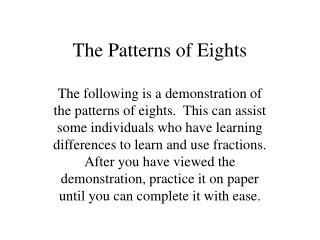DownloadDownload PresentationThe Patterns of Eights

# The Patterns of Eights

Download Presentation## The Patterns of Eights

- - - - - - - - - - - - - - - - - - - - - - - - - - - E N D - - - - - - - - - - - - - - - - - - - - - - - - - - -
##### Presentation Transcript

1. The Patterns of Eights The following is a demonstration of the patterns of eights. This can assist some individuals who have learning differences to learn and use fractions. After you have viewed the demonstration, practice it on paper until you can complete it with ease.

2. There are four patterns to remember. • 1. Write the numbers 1 to 8 without the 4. • 2. Place a 5 behind the 2 and the 7. • 3. Place 25 behind the 1, 75 behind the 3, 25 behind the 6, 75 behind the 8. • 4. Place a decimal point in front of each number.

3. 1

4. 21

5. 321

6. Skip 4

7. 5321

8. 65321

9. 765321

10. 8765321

11. 87653251

12. 875653251

13. 87565325125

14. 8756537525125

15. 875625537525125

16. 87575625537525125

17. .875.75.625.5.375.25.125

18. .875 = 7/8.75 = 3/4.625 = 5/8.5 = 1/2.375 = 3/8.25 = 1/4.125 = 1/8.875 = 7/8.75 = 3/4.625 = 5/8.5 = 1/2.375 = 3/8.25 = 1/4.125 = 1/8